186

8

13

8

# Latexify.jl

This is a package for generating LaTeX maths from julia objects.

This package utilises Julias homoiconicity to convert expressions to LaTeX-formatted strings. Latexify.jl supplies functionalities for converting a range of different Julia objects, including:

• Expressions,
• Strings,
• Numbers (including rationals and complex),
• Symbolic expressions from SymEngine.jl,
• ParameterizedFunctions and ReactionNetworks from DifferentialEquations.jl

as well as arrays or dicts of supported types.

### Examples

#### latexifying expressions

using Latexify
ex = :(x/(y+x)^2)
latexify(ex)


This generates a LaTeXString (from LaTeXStrings.jl) which, when printed looks like:

$\frac{x}{\left( y + x \right)^{2}}$


And when this LaTeXString is displayed in an environment which supports the tex/latex MIME type (Jupyter notebooks, Jupyterlab and Hydrogen for Atom) it will automatically render as: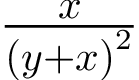#### latexifying other things

Latexify.jl is equipped to convert a whole range of types to latex formatted maths. This includes primitive types such as Symbols and Complex, but also of containers such as Arrays and Dicts.

using Latexify
print(latexify("x+y/(b-2)^2"))


outputs:

$x + \frac{y}{\left( b - 2 \right)^{2}}$

arr = ["x/y" 3//7 2+3im; 1 :P_x :(gamma(3))]
latexify(arr)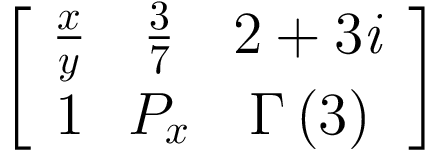The GitHub website does not really support rendering of equations in the README file, so I therefore refer you to the documentation for more info/examples.

### Use with DifferentialEquations.jl

The DifferentialEquations.jl suite has some nifty tools for generating differential equations. One of them is ParameterizedFunctions which allows you to type in an ODE in something which looks very much like just plain mathematics. The ability to latexify such ODEs is pretty much what lured me to create this package.

using Parameterizedfunctions
using Latexify

f = @ode_def positiveFeedback begin
dx = v*y^n/(k^n+y^n) - x
dy = x/(k_2+x) - y
end v n k k_2

latexify(f)


outputs: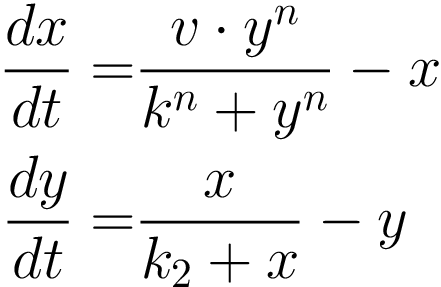DiffEqBiological.jl provides another cool domain-specific language which allows you to generate equations using a chemical arrow notation.

using DiffEqBiological
using Latexify

rn = @reaction_network demoNetwork begin
(r_bind, r_unbind), A + B ↔ C
Hill(C, v, k, n), 0 --> X
d_x, X --> 0
end r_bind r_unbind v k n d_x

latexify(rn)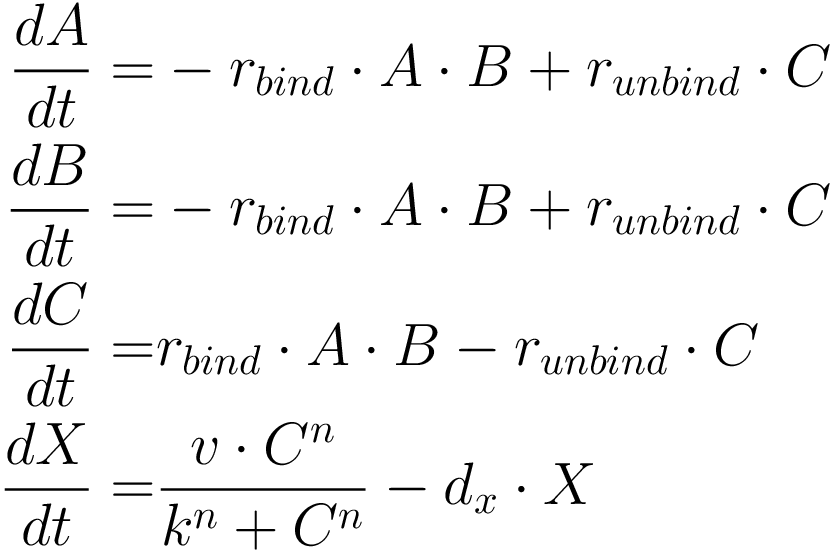Or you can output the arrow notation directly to latex:

latexify(rn; env=:arrow)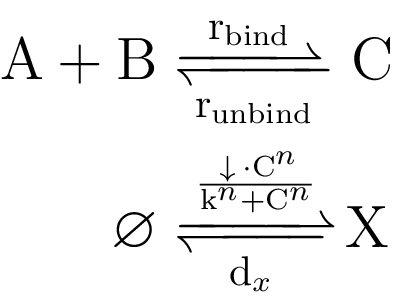There are more stuff that you can do, but for that I will refer you to the docs.

## Convenience functions

• copy_to_clipboard(::Bool), toggle automatic copying of the resulting LaTeX code to the clipboard (default is false).
• auto_display(::Bool) toggles automatic display of your output, even if it is not the last command to have run.
• set_default(; kwargs...), set your own default kwargs for your Julia session. This is not to be used within a package since the effect is global.
• reset_default(; kwargs...), reset the changes you made with the above command.
• get_default(; kwargs...), view the changes you have made to the default kwargs.

## Installation

This package is registered with METADATA.jl, so to install it you can just run

Pkg.add("Latexify")


## Further information

For further information see the docs.

## Contributing

I would be happy to receive feedback, suggestions, and help with improving this package. Please feel free to open an issue or a PR.

10/19/2017

2 days ago

394 commits